Terms & Symbols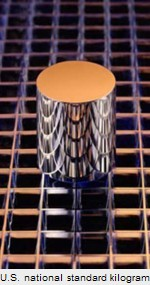To show clearly the consequences of the quantum medium that explain WHY the phenomena we've been discussing occur, we must introduce new terms and symbols that are not part of the lexicon of orthodox physics theory. When these terms and symbols are first introduced and defined, this will be done with bold type. The Glossary contains these and other terms and symbols and it can be opened by clicking the Glossary button that appears on every page.

The absolute speed of light (ca) is the speed of light through the quantum medium (qm) when the light is not impeded by matter or by the proximity of large concentrations of mass/energy. This speed of light is 299,792,458 meters per second which, for simplicity, is rounded to 300,000,000. The symbol c means the virtual constant speed of light observed by observers(c). Large and small numbers such as 300,000,000 and .000,000,001 will generally be written as 3E8 and 1E−9, where E means "times 10 to the power." The math symbols, · and /, mean "times" and "divided by," and ()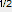means "square root" of what is inside ().

The absolute velocity (va) of a body or reference frame is its velocity through the qm, and the velocity is usually specified in terms of ca. Therefore, a body with an absolute velocity of va=.5 ca has a velocity through the qm of half the speed of light through the medium.

An absolute second (sa) is 1 second according to clocks at rest in the qm. A virtual second (s) is 1 second according to a clock moving through the medium. It will soon be apparent why 1 s is a longer time duration than 1 sa. The symbol ta designates absolute time before or after a particular event such as a light turning on. Thus ta=−1 sa and ta=+1 sa mean one absolute second before and after a specified event.

An absolute light-second (LS) is the distance that light travels through the qm in 1 sa and a virtual light-second (ls) is the distance between two points in an inertial reference frame where a round-trip light signal between the two points takes 2 s according to clocks in the reference frame. An absolute meter (ma) is 1 LS/3E8 and a virtual meter (m) is ls/3E8. It will be seen that the length of a standard meter bar depends on the bar's orientation in the cosmos. Due to Earth's rotation and revolution, the bar has periodic daily and yearly variations in length, just as a standard kilogram (left) has small daily and yearly variations in mass.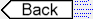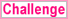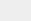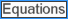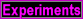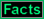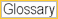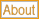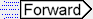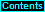To page: 1 2 3 3a 4 5 6 7 8 9 10 11 12 13 14 15 16 17 18 19 20 21 22 23 24 25 26 27 28 29 29a 30 31 32